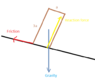# Equivalence of tipping conditions on an inclined plane

• ChessEnthusiast

#### ChessEnthusiast

We have a cube on an inclined plane.
The tipping condition is the presence of an unbalanced torque relative to the center of mass (contributing forces are: the normal force and the force of friction).

However, is this conditions equivalent to the previous one:
The line of action of the force of gravity points outside the base of the cube AND the force of friction is large enough to prevent the cube from sliding?

If not, it it easy to think of a simple counterexample?

An object can slide without tipping over.

Let's say I have a cuboid with sides $a$, $a$ and $3a$.
The distance of the line of action of the force of friction from the center of gravity is $\frac 3 2 a$
As for the reaction force, the maximal distance will be $\frac a 2$.
We are considering the static case. Therefore, the force of friction:
$$F_f = mg \sin(\theta)$$
And the normal force
$$F_n = mg \cos(\theta)$$
In the language of torques:
$$\frac{3}{2}a mg \sin(\theta) = \frac{1}{2}a mg \cos(\theta)$$
$$3 sin(\theta) = cos(\theta)$$
$$\tan(\theta) = \frac{1}{3}$$
$$\theta = 18 \deg$$
This is exactly the same angle that I get when I try to work out the situation when the force of gravity is getting outside the base of the cuboid (once again, I'm considering the static case).
Is it a coincidence?

#### Attachments

•cube3.PNG
2.9 KB · Views: 407
We are considering the static case.
You cannot just assume this. You need to verify that you can get a friction force that is large enough.

Suppose that I know that the object will not slide - is it, then, sufficient to find the angle that causes the line of action to leave the base of the cuboid?